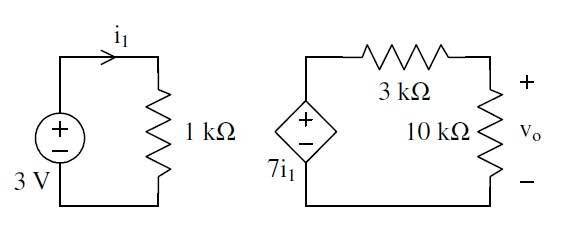# Output voltage of a circuit

• Engineering

## Homework Statement

Find the output voltage vo in the following circuitV = IR

## The Attempt at a Solution

Ok well I've been going over a couple more questions before my exam in introductory circuit analysis and here's another one I've never really seen before. This may sound stupid but the fact that it's like two different circuits is kind of confusing me.

Could someone at least point me in the right direction towards solving this problem? Really appreciate it everyone, thanks.

berkeman
Mentor

## Homework Statement

Find the output voltage vo in the following circuitV = IR

## The Attempt at a Solution

Ok well I've been going over a couple more questions before my exam in introductory circuit analysis and here's another one I've never really seen before. This may sound stupid but the fact that it's like two different circuits is kind of confusing me.

Could someone at least point me in the right direction towards solving this problem? Really appreciate it everyone, thanks.

It's all one circuit, actually. The left part defines the current, which is then used in the right circuit's "current controlled voltage source". So solve for the current in the left circuit first, and then use that current to set the voltage of the voltage source, and then solve for the voltage divider in the right circuit to get the output voltage.

Makes sense?

Last edited:
It's all one circuit, actually. The left part defines the current, which is then used in the right circuit's "current controlled voltage source". So solve for the current in the left circuit first, and then use that current to set the voltage of the voltage source, and then solve for the voltage divider in the right circuit to get the output voltage.

Makes sense?

Perfect sense, thanks!

EDIT: Just did the calculation and got the correct answer. Thanks for the help again

Last edited: